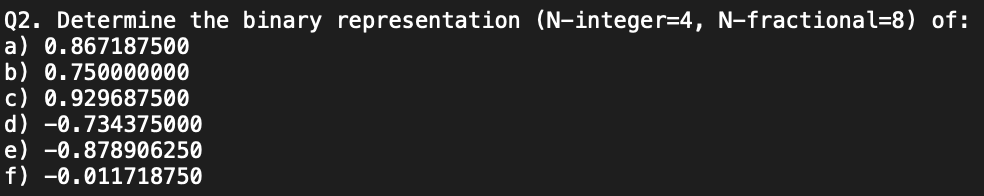Home / Expert Answers / Other Math / q2-determine-the-binary-representation-n-integer-4-n-fractional-8-of-a-0-867187500-b-0-75000-pa772

# (Solved): Q2. Determine the binary representation (N-integer =4,N-fractional=8) of: a) 0.867187500 b) 0.75000 ...Q2. Determine the binary representation (N-integer -fractional=8) of: a) 0.867187500 b) 0.750000000 c) 0.929687500 d) -0.734375000 e) -0.878906250 f) -0.011718750

We have an Answer from Expert

Given, N Integer= 4 & N-Fractional = 8

Steps to convert a Fractional representation to a Binary

Multiply by 2
Take Decimal as the Digit
Take the fraction as the starting point of the next step
Repeat this process until you get "0"or a periodic Number
Now read the number starting form the top

We have an Answer from Expert